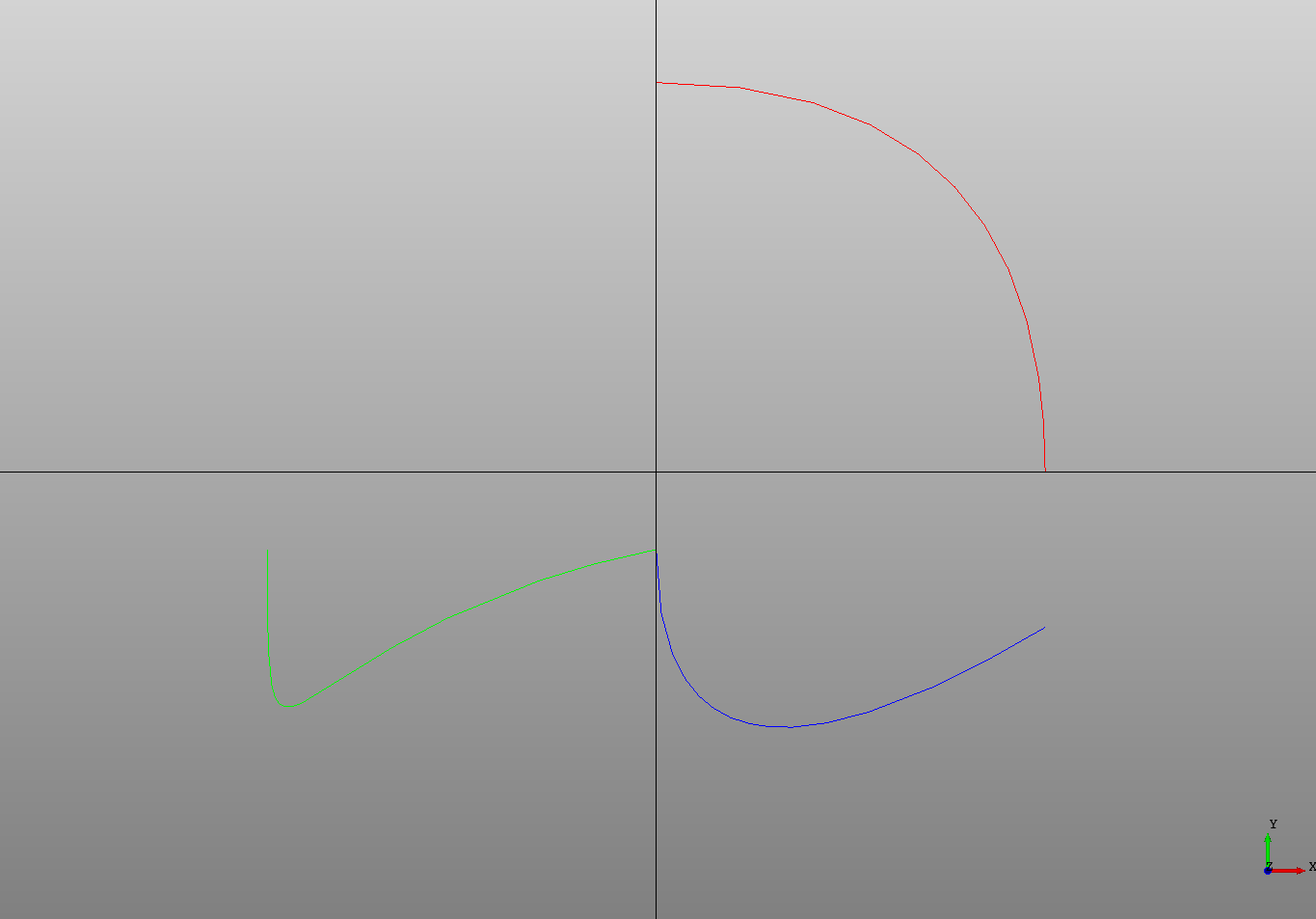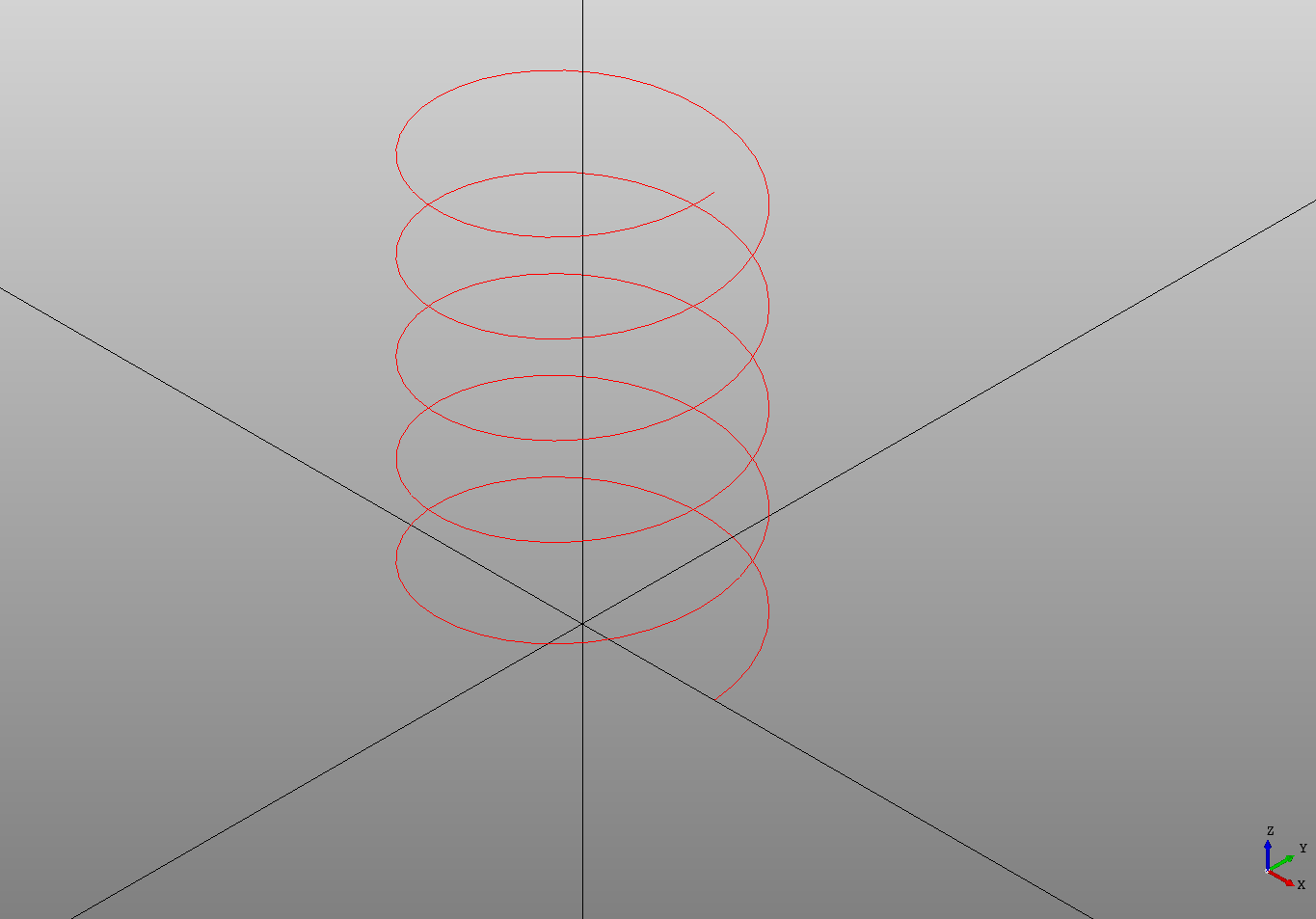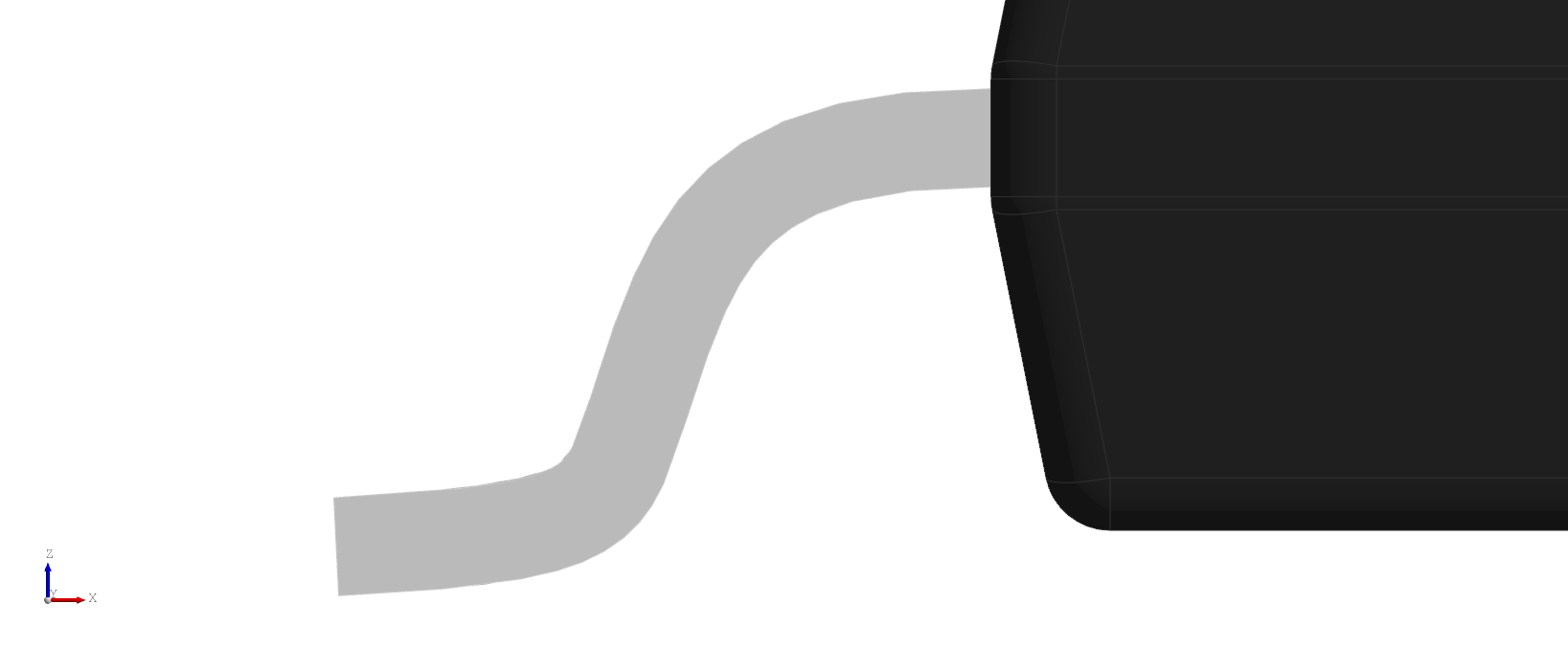## Beziers

DeclaraCAD supports both quadratic and cubic `Bezier` curves which can be defined by a set of three or four points respectively.

``````# Created in DeclaraCAD
from math import pi

enamldef Assembly(Part):
Bezier: b1: # An arc
color = 'red'
points = [(0, 5), (5, 5), (5, 0)]

Bezier: b2:
color = 'blue'
points = [(0, -1), (0, -5), (5, -2)]

Bezier: b3:
color = 'lime'
points = [(-5, -1), (-5, -5), (-5, -2), (0, -1)]

# For reference
Line: xaxis:
color = 'black'
direction = (1, 0)
Line: yaxis:
color = 'black'
direction = (0, 1)
``````## BSplines

DeclaraCAD supports `BSpline` cruves which are created by interpolating all the `points`.

These can be used with a little bit of trig to create a helix:

``````# Created in DeclaraCAD
from math import pi, sin, cos

enamldef Assembly(Part):
BSpline: bspline:
attr turns = 10
attr r = 3
color = 'red'
points = [
(r*cos(pi/4*i), r*sin(pi/4*i), i/4)
for i in range(turns*4+1)
]

# For reference
Line: xaxis:
color = 'black'
direction = (1, 0)
Line: yaxis:
color = 'black'
direction = (0, 1)
Line: zaxis:
color = 'black'
direction = (0, 0, 1)
``````A list of `tangents` can be specified for bsplines. If only two tangents are provided they will be interpreted as the start and stop tangents, otherwise one tangent should be given for each point. This can be used to ensure continuity.

``````        BSpline: spline:
# Path of leg from PCB surface to package
# zero'd at the package entry point.
tangents = [
(1, 0, 0),
(1, 0, -0.05),

]
points = [
(0, 0, offset),
(width/2-thickness/2, 0, offset-thickness),
(width/2+thickness-0.1, 0, 0.25),
(width, 0, thickness/2),
]
``````